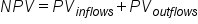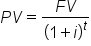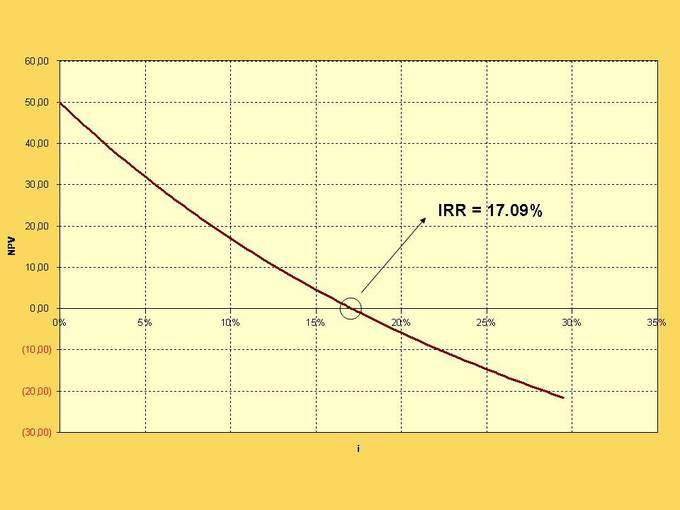+

# Net Present Value

Author: Sophia Tutorial
##### Description:

Identify the advantages and disadvantages of NPV as a capital budget method.

(more)### Developing Effective Teams

*No strings attached. This college course is 100% free and is worth 1 semester credit.

37 Sophia partners guarantee credit transfer.

299 Institutions have accepted or given pre-approval for credit transfer.

* The American Council on Education's College Credit Recommendation Service (ACE Credit®) has evaluated and recommended college credit for 33 of Sophia’s online courses. Many different colleges and universities consider ACE CREDIT recommendations in determining the applicability to their course and degree programs.

Tutorial

what's covered
In this lesson, you will learn about the advantages and disadvantages of net present value as a capital budget method. Specifically, this lesson will cover:
1. Defining the Net Present Value
2. Interpreting the Net Present Value
3. Advantages of the Net Present Value
4. Disadvanatages of the Net Present Value
5. Net Present Value Profiles

## 1. Defining the Net Present Value

Every investment includes cash outflows and cash inflows. There is the cash that is required to make the investment and, hopefully, the return. In order to see whether the cash outflows are less than the cash inflows (i.e., the investment earns a positive return), the investor aggregates the cash flows. Since cash flows occur over a period of time, the investor knows that due to the time value of money, each cash flow has a certain value today. Thus, in order to sum the cash inflows and outflows, each cash flow must be discounted to a common point in time.

EXAMPLE

Before purchasing a new airplane, airlines evaluate the NPV of the plan by calculating the PV of the revenue it can earn from it and the PV of its cost (e.g., purchase cost, maintenance, fuel, etc.).

The net present value (NPV) is simply the sum of the present values (PVs) of all the outflows and inflows:

formula

Net Present Valuehint
Don’t forget that inflows and outflows have opposite signs. Cash inflows, such as coupon payments or the repayment of principal on a bond, have a positive sign while cash outflows, such as the money used to purchase the investment, have a negative sign.

Also, recall that PV is found by the following formula:

formula

Present ValueIn this formula, FV is the future value, or the size of each cash flow, i is the discount rate, and t is the number of periods between the present and future. The PV of multiple cash flows is simply the sum of the PVs for each cash flow.

The accurate calculation of NPV relies on knowing the amount of each cash flow and when each will occur. For securities like bonds, this is an easy requirement to meet. The bond clearly states when each coupon payment will occur, the size of each payment, when the principal will be repaid, and the cost of the bond. For other investments, this is not so simple to determine. When a new piece of machinery is purchased, for example, the investor (the purchasing company) has to estimate the size and occurrence of maintenance costs as well as the size and occurrence of the revenues generated by the machine.

Another important variable involved with calculating NPV is the discount rate. The most commonly used rate for this is the weighted average cost of capital. There is some disagreement around the use of this rate, as there are some that believe it does not adequately account for risk and other factors.

terms to know

Cash Outflow
Any cash that is spent or invested by the investor.
Cash Inflow
Cash that is received by the investor, such as dividends paid on a stock owned by the investor.

## 2. Interpreting the Net Present Value

The sign of NPV can explain a lot about whether the investment is good or not:

• NPV > 0: The PV of the inflows is greater than the PV of the outflows. The money earned on the investment is worth more today than the costs; therefore, it is a good investment.
• NPV = 0: The PV of the inflows is equal to the PV of the outflows. There is no difference in value between the value of the money earned and the money invested.
• NPV < 0: The PV of the inflows is less than the PV of the outflows. The money earned on the investment is worth less today than the costs; therefore, it is a bad investment.
In theory, an investor should make any investment with a positive NPV, which means the investment is making money. Similarly, an investor should refuse any option that has a negative NPV because it only subtracts from the value. When faced with multiple investment choices, the investor should always choose the option with the highest NPV. This is only true if the option with the highest NPV is not negative. If all the investment options have negative NPVs, none should be undertaken.

The decision is rarely that cut and dry, however. The NPV is only as good as the inputs. The NPV depends on knowing the discount rate, when each cash flow will occur, and the size of each flow. Cash flows may not be guaranteed in size or when they occur, and the discount rate may be hard to determine. Any inaccuracies and the NPV will be affected, too.

## 3. Advantages of the Net Present Value

Calculating the NPV is a way investors determine how attractive a potential investment is. Since it essentially determines the present value of the gain or loss of an investment, it is easy to understand and is a great decision-making tool. In theory, an investor should undertake positive NPV investments, and never undertake negative NPV investments. Thus, NPV makes the decision-making process relatively straight forward.

Another advantage of the NPV method is that it allows for easy comparisons of potential investments. As long as the NPV of all options is taken at the same point in time, the investor can compare the magnitude of each option. When presented with the NPVs of multiple options, the investor will simply choose the option with the highest NPV because it will provide the most added value for the firm. However, if none of the options has a positive NPV, the investor will not choose any of them; none of the investments will add value to the firm, so the firm is better off not investing.

Furthermore, NPV is customizable so that it accurately reflects the financial concerns and demands of the firm.

EXAMPLE

The discount rate can be adjusted to reflect things such as risk, opportunity cost, and changing yield curve premiums on long-term debt.

## 4. Disadvantages of the Net Present Value

There are a number of disadvantages to NPV. NPV is still commonly used, but firms will also use other metrics before making investment decisions.

The first disadvantage is that NPV is only as accurate as the inputted information. It requires that the investor know the exact discount rate, the size of each cash flow, and when each cash flow will occur. Often, this is impossible to determine.

EXAMPLE

When developing a new product, such as a new medicine, the NPV is based on estimates of costs and revenues. The cost of developing the drug is unknown and the revenues from the sale of the drug can be hard to estimate, especially many years in the future.

Furthermore, the NPV is only useful for comparing projects at the same time; it does not fully build in opportunity cost. For instance, the day after the company makes a decision about which investment to undertake based on NPV, it may discover there is a new option that offers a superior NPV. Thus, investors don’t simply pick the option with the highest NPV; they may pass on all options because they think another better option may come along in the future. NPV does not build in the opportunity cost of not having the capital to spend on future investment options.

Another issue with relying on NPV is that it does not provide an overall picture of the gain or loss of executing a certain project. To see a percentage gain relative to the investments for the project, internal rate of return (IRR) or other efficiency measures are used as a complement to NPV.

term to know

Opportunity Cost
The cost of an opportunity forgone (and the loss of the benefits that could be received from that opportunity); the most valuable forgone alternative.

## 5. Net Present Value Profiles

The NPV calculation involves discounting all cash flows to the present based on an assumed discount rate. When the discount rate is large, there are larger differences between PV and FV for each cash flow than when the discount rate is small. Thus, when discount rates are large, cash flows further in the future affect NPV less than when the rates are small. Conversely, a low discount rate means that NPV is affected more by the cash flows that occur further in the future.

The relationship between NPV and the discount rate used is calculated in a chart called an NPV profile. The independent variable is the discount rate and the dependent variable is the NPV. The NPV profile assumes that all cash flows are discounted at the same rate.The NPV profile usually shows an inverse relationship between the discount rate and the NPV. While this is not necessarily true for all investments, it can happen because outflows generally occur before the inflows. A higher discount rate places more emphasis on earlier cash flows, which are generally the outflows. When the value of the outflows is greater than the inflows, the NPV is negative.

A special discount rate is highlighted in the IRR, which stands for Internal Rate of Return. It is the discount rate at which the NPV is equal to zero and is the discount rate at which the value of the cash inflows equals the value of the cash outflows.

summary
In this lesson, you learned more about the definition of net present value, which is the sum of the present values of all the estimated cash inflows and outflows of a project. Net present value is a common capital budgeting tool. Interpreting the net present value is relatively easy; investments with a positive NPV should be approved and those with a negative NPV should not. This simplicity is an advantage of net present value, as is its use in comparing multiple projects. However, there are disadvantages of net present value as well, such as the fact that it relies on cash flow estimates that can be difficult to forecast. Finally, you looked at net present value profiles, which chart the inverse relationship between NPV and the discount rate.

Best of luck in your learning!

Source: THIS CONTENT HAS BEEN ADAPTED FROM LUMEN LEARNING'S “Net Present Value” TUTORIAL.

Terms to Know
Cash Inflow

Cash that is received by the investor, such as dividends paid on a stock owned by the investor.

Cash Outflow

Any cash that is spent or invested by the investor.

Opportunity Cost

The cost of an opportunity forgone (and the loss of the benefits that could be received from that opportunity); the most valuable forgone alternative.

Formulas to Know
Net Present ValuePresent ValueRating